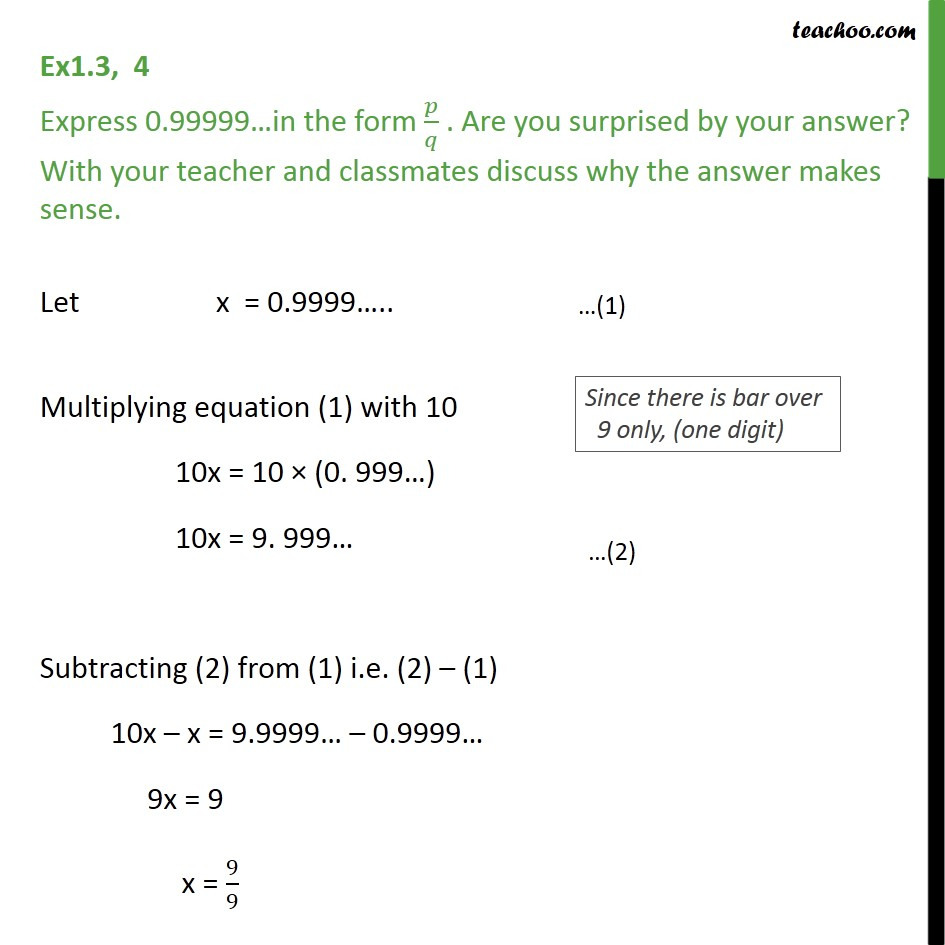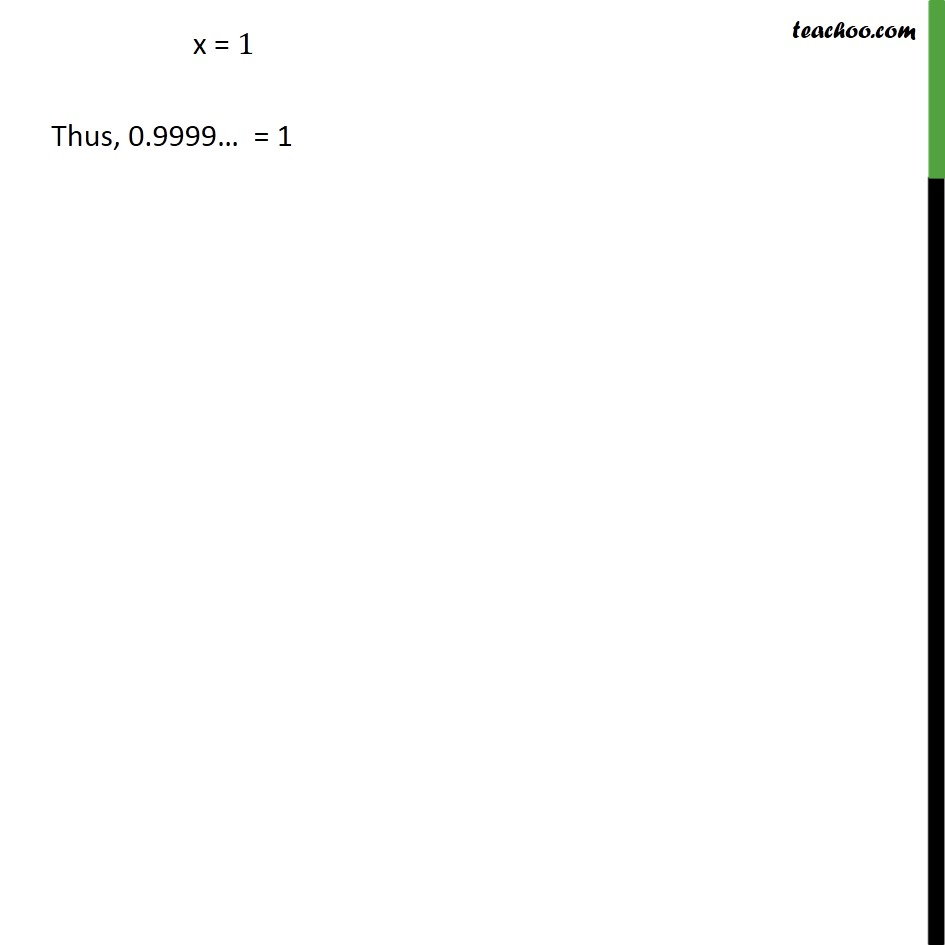1. Chapter 1 Class 9 Number Systems
2. Concept wise
3. Expressing decimal in p/q

Transcript

Ex1.3, 4 Express 0.99999…in the form 𝑝/𝑞 . Are you surprised by your answer? With your teacher and classmates discuss why the answer makes sense. Let x = 0.9999….. Multiplying equation (1) with 10 10x = 10 × (0. 999…) 10x = 9. 999… Subtracting (2) from (1) i.e. (2) – (1) 10x – x = 9.9999… – 0.9999… 9x = 9 x = 9/9 x = 1 Thus, 0.9999… = 1

Expressing decimal in p/q